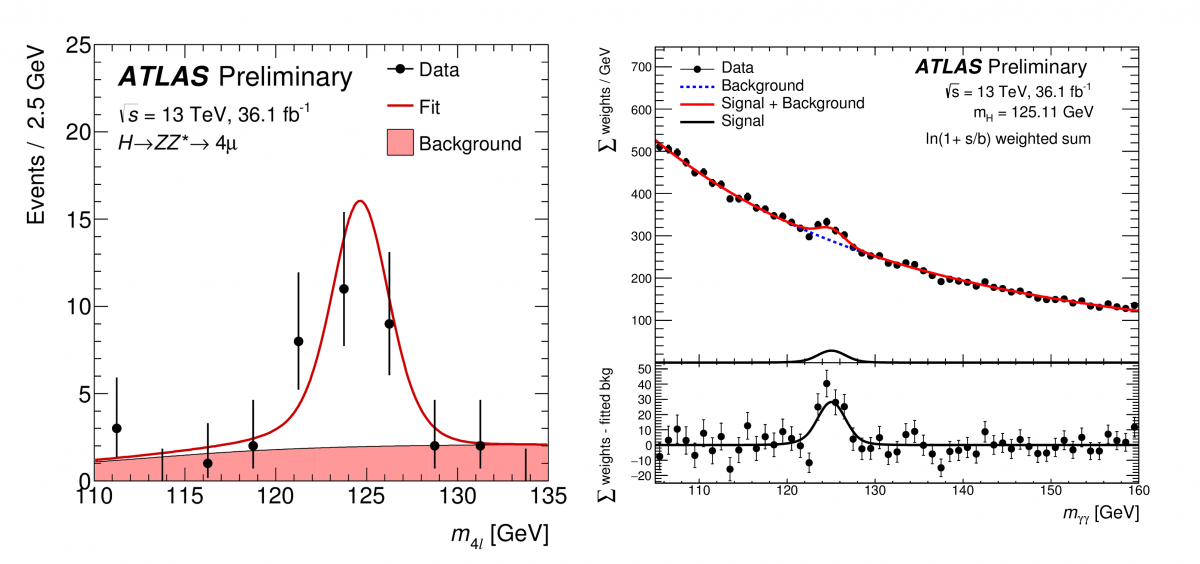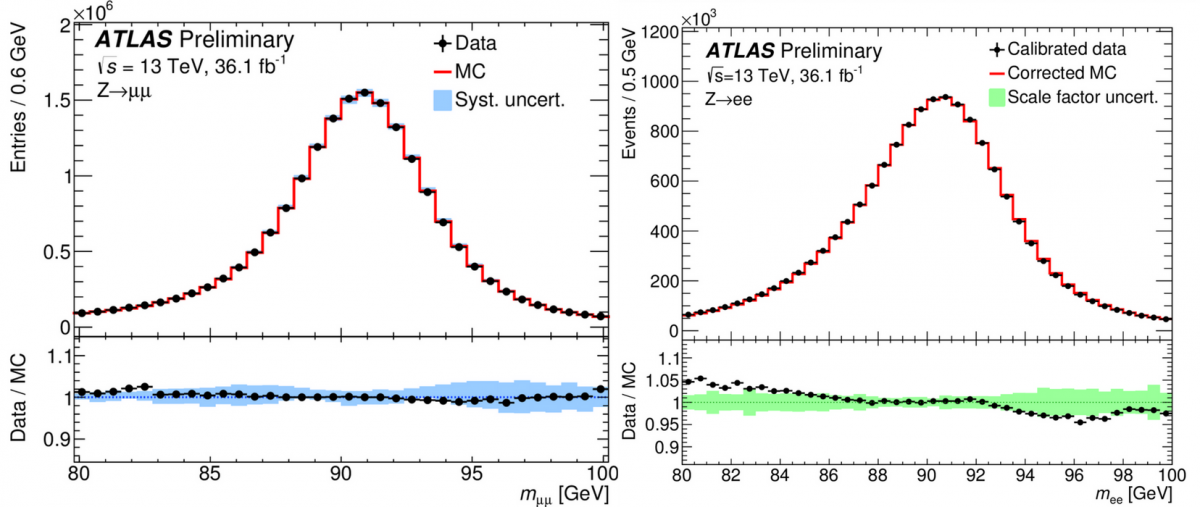Tags:

# New ATLAS measurement of the Higgs Boson mass

6th July 2017 | ByFigure 1: The invariant mass spectrum of the four leptons selected in measurement of H → ZZ* → 4l (left) and of the two photons in the measurement of H → γγ (right). The red line is the fit to the data points where the fit model includes the expected shape for a Higgs Boson as well as the backgrounds. The backgrounds are mostly from the (non-resonant) qq → ZZ* (left) and qq → γγ (right) production in proton-proton collisions. The lower plot on the right has the background subtracted from the upper plot. One can clearly see the fits both peak at a mass of about 125 GeV. (Image: ATLAS Collaboration/CERN)

The ATLAS collaboration has released a new preliminary measurement of the Higgs boson mass using 2015 and 2016 LHC data. The number of recorded Higgs boson events has more than tripled since the first measurement of the Higgs boson was released, using 2011/2012 data. An improved precision in the measurement of the Higgs boson mass has been made possible by both the increased collision energy of 13 TeV and improved collision rate.

The new ATLAS result looks at the Higgs boson decaying to four leptons (H → ZZ* → 4l), where leptons can be pairs of muons or electrons, and to two photons (H → γγ). These are the best decay channels to measure the Higgs mass as all of the decay particles can be measured by the ATLAS detector with high precision. The four-lepton channel has a clear and clean signature due to its small background contamination, making it ideal for study despite its rarity. The two-photon channel, while more common, features a large background. The measurement of the Higgs boson mass, reconstructed from the energy and momentum of these decay products, is shown in Figure 1.Figure 2: The di-muon invariant mass distribution for Z → μ+μ- decays (left) and the di-electron invariant mass distribution for Z → e+e- decays (right). Both data and Monte Carlo simulation are shown. The bottom plots show the ratio of the two as well as the uncertainty bands.(Image: ATLAS Collaboration/CERN)

The key to precise Higgs boson mass measurements lies in the resolution and calibration of the ATLAS detector. Many different parts of the detector are used: the muon momentum is measured in the Inner Detector and the Muon Spectrometer, while the electron and photon energy is reconstructed in the Liquid Argon electromagnetic calorimeter. Understanding the alignment of the tracking detectors provides the precision of the muon momentum, and the calibration of the calorimeter response is key for electrons and photons. The uncertainty of the muon momentum is known to 0.1–0.5%, depending on the position of the muon, while the uncertainty on the electron (photon) energy scale is 0.02–0.8% (0.4–0.8%) depending on their energy and position. The quality of the alignment and calibration of the data can be seen in Figure 2, where the abundant Z bosons decay into two muons and into two electrons.

### The key to precise Higgs boson mass measurements lies in the resolution and calibration of the ATLAS detector.

The results are summarized in Figure 3, where the individual measurements for the two channels are given as well as their combination: 124.98 ± 0.28 GeV. The LHC Run 1 combined measurement is also provided for comparison. The four-lepton measurement remains dominated by its statistical uncertainty due to the small number of events, and the two-photon measurement has a larger systematic uncertainty from the photon energy calibration. From the grey band, corresponding to the uncertainty of the combined measurement, the agreement between the four-lepton and two-photon measurements, as well as with the Run 1 result, is excellent.Figure 3: The summary of the Higgs boson mass measurements from the individual and combined analyses, compared to the combined Run 1 measurement by ATLAS and CMS. The systematic (magenta-shaded bands), statistical (yellow-shaded bands), and total (black error bars) uncertainties are indicated. The (red) vertical line and corresponding (grey) shaded column indicate the central value and the total uncertainty of the combined measurement, respectively. (Image: ATLAS Collaboration/CERN)

### Links:

1. Measurement of the Higgs boson mass in the H→ZZ∗→4ℓ and H→γγ channels with 13 TeV proton-proton collisions using the ATLAS detector (ATLAS-CONF-2017-046).
2. EPS 2017 presentation by Karolos Potamianos: Measurement of the Standard Model Higgs boson mass in the diphoton and 4l decay channels using the ATLAS detector
3. Combined Measurement of the Higgs Boson mass in proton-proton collisions at 7 and 8 TeV with the ATLAS and CMS Experiments (Phys. Rev. Lett. 114 (2015) 191803 and arXiv: 1503.07589)
4. See also the full lists of ATLAS Conference Notes and ATLAS Physics Papers.